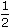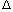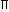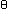# Aptitude - Area

### Exercise :: Area - Important Formulas

FUNDAMENTAL CONCEPTS

1. Results on Triangles:

1. Sum of the angles of a triangle is 180°.

2. The sum of any two sides of a triangle is greater than the third side.

3. Pythagoras Theorem:

In a right-angled triangle, (Hypotenuse)2 = (Base)2 + (Height)2.

4. The line joining the mid-point of a side of a triangle to the opposite vertex is called the median.

5. The point where the three medians of a triangle meet, is called centroid. The centroid divided each of the medians in the ratio 2 : 1.

6. In an isosceles triangle, the altitude from the vertex bisects the base.

7. The median of a triangle divides it into two triangles of the same area.

8. The area of the triangle formed by joining the mid-points of the sides of a given triangle is one-fourth of the area of the given triangle.

1. The diagonals of a parallelogram bisect each other.

2. Each diagonal of a parallelogram divides it into triangles of the same area.

3. The diagonals of a rectangle are equal and bisect each other.

4. The diagonals of a square are equal and bisect each other at right angles.

5. The diagonals of a rhombus are unequal and bisect each other at right angles.

6. A parallelogram and a rectangle on the same base and between the same parallels are equal in area.

7. Of all the parallelogram of given sides, the parallelogram which is a rectangle has the greatest area.

IMPORTANT FORMULAE

1. 1.   Area of a rectangle = (Length x Breadth).Length =Areaand Breadth =Area. Breadth Length

2.   Perimeter of a rectangle = 2(Length + Breadth).

2. Area of a square = (side)2 =(diagonal)2.

3. Area of 4 walls of a room = 2 (Length + Breadth) x Height.

4. 1.   Area of a triangle =x Base x Height.

2.   Area of a triangle = s(s-a)(s-b)(s-c)
where a, b, c are the sides of the triangle and s =(a + b + c).

 3.   Area of an equilateral triangle = 3 x (side)2. 4

 4.   Radius of incircle of an equilateral triangle of side a = a . 23

 5.   Radius of circumcircle of an equilateral triangle of side a = a . 3

 6.   Radius of incircle of a triangle of areaand semi-perimeter r =. s

5. 1.   Area of parallelogram = (Base x Height).

2.   Area of a rhombus =x (Product of diagonals).

3.   Area of a trapezium =x (sum of parallel sides) x distance between them.

6. 1.   Area of a circle =R2, where R is the radius.

2.   Circumference of a circle = 2R.

 3.   Length of an arc = 2R, whereis the central angle. 360

 4.   Area of a sector = 1 (arc x R) =R2. 2 360

7. 1.   Circumference of a semi-circle =R.

 2.   Area of semi-circle =R2 . 2